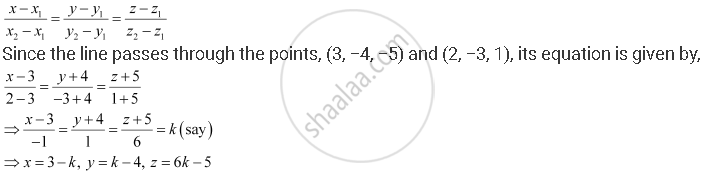Share

# Find the Coordinates of the Point Where the Line Through (3, ­−4, −5) and (2, − 3, 1) Crosses the Plane 2x + Y + Z = 7). - CBSE (Commerce) Class 12 - Mathematics

ConceptPlane Equation of a Plane in Normal Form

#### Question

Find the coordinates of the point where the line through (3, ­−4, −5) and (2, − 3, 1) crosses the plane 2x + z = 7).

#### Solution

It is known that the equation of the line through the points, (x1y1z1) and (x2y2z2), isTherefore, any point on the line is of the form (3 − kk − 4, 6k − 5).

This point lies on the plane, 2x + y + z = 7

∴ 2 (3 − k) + (k − 4) + (6k − 5) = 7

=> 5k - 3 =7

=> k = 2

Hence, the coordinates of the required point are (3 − 2, 2 − 4, 6 × 2 − 5) i.e.,

(1, −2, 7).

Is there an error in this question or solution?

#### APPEARS IN

NCERT Solution for Mathematics Textbook for Class 12 (2018 to Current)
Chapter 11: Three Dimensional Geometry
Q: 12 | Page no. 498
Solution Find the Coordinates of the Point Where the Line Through (3, ­−4, −5) and (2, − 3, 1) Crosses the Plane 2x + Y + Z = 7). Concept: Plane - Equation of a Plane in Normal Form.
S